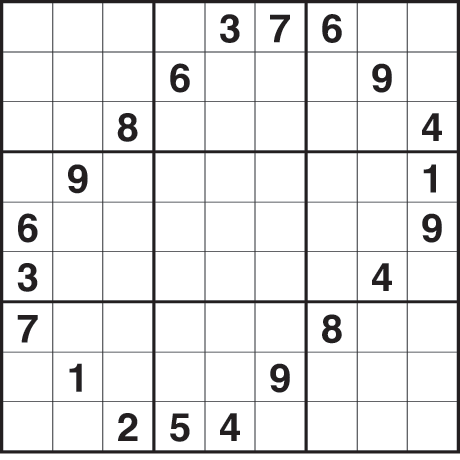# The P vs NP problem

ICYMI. Enjoy! -AB

***

### Checking is easier than finding?

Finding a solution to a problem should be harder than checking that a solution is correct. That seems obvious if you’ve ever solved a sudoku puzzle, or solved a Rubik’s cube, or played a game of chess. But that statement, however intuitive looking, leads us directly to one of the deepest mathematical questions posed by our civilization.

Non-deterministic polynomial time (or NP) problems are ones where you can easily verify an answer to be correct. In contrast, polynomial time (or P) problems are those
where finding the answer is easy.  If you can find the answer, then you have checked it to be correct, so every problem in P is also in NP.  We say P is a subclass of NP.  However, we don’t know whether there is any particular problem in NP that is not in P,  and that is what we call the P vs NP problem.

The P vs NP problem is one of the most central unsolved problems in mathematics and theoretical computer science. There is even a Clay Millennium Prize offering one million dollars for its solution. However, there are likely much easier ways to become a millionaire than solving P vs NP!

There was a buzz recently when someone purported to solve it on arXiv, although a gap in the proof was quickly found. Given the recent interest in the problem and its central place in mathematics, I discuss it in this week’s blog.

### Sudoku

If you are given a filled-in Sudoku puzzle in a newspaper, you might chuck it in the bin. But if you wanted, you could scan through the rows, columns, and 3 x 3 cells to tell it is a valid solution: each of 1, 2, … , 9 must occur exactly once. That is analogous to NP, as it is relatively easy to check if a solution is correct.

However, if I give you a partially filled Sudoku puzzle, it can be tough to find the solution. That’s the joy and challenge of this kind of puzzle. So being in NP doesn’t immediately seem to imply the problem has an easy to find a solution. That intuition is at the heart of the P vs NP problem.Quick, what is the solution?

### Precise formulation

To understand P and NP more precisely, we need to better understand algorithms and measure their speed. Problems are posed with some fixed input and output either YES or NO. The input is a given length, say n, where n is a positive integer. This represents the number of bits it takes to express the input. An algorithm is a method or procedure for solving a problem. Algorithms provide instructions at every step in a computation and must terminate. The steps could be seconds, milliseconds, or some other fixed interval of time that depends on your problem.

The complexity of a problem is the minimum worst-case running time over all possible algorithms solving the problem as a function of the length of the input.  In other words, it measures how long it takes to solve the problem with the fastest algorithm but with the worst case input.

A problem is solvable in polynomial time if given an input of length n, its complexity is bounded above by the polynomial function nm for some non-negative integer m. The set of all problems solvable in polynomial time is denoted by P.

Being in P doesn’t necessarily mean the problem is feasible. For example, if it takes n1000 steps to run the algorithm, then it will be hopelessly slow even for small n. Non-deterministic polynomial (or NP) problems are ones where you can check if the YES answer is correct in polynomial time.

### NP-hardness

A problem is NPhard if a polynomial-time algorithm for it would imply a polynomial-time algorithm for every problem in NP. Hence, if an NP-hard problem were in P, then NP. The NP-hard problems are at least as hard as any problem in NP. An NPcomplete problem is one which is NP-hard and in NP.A Hamilton cycle in red.

A classic NP-complete problem is finding a Hamilton cycle.  For this, suppose we have a network of cities connected by roads. The problem is to visit each city using roads (no planes allowed!) and come back to the start.  This seems easy for a small number of cities, but if you were given hundreds of them then it becomes hard. Thousands of other such NP-complete problems are known arising in graph theory, number theory, geometry, and other areas of mathematics and computer science.

### What’s the answer, really?

If I were to bet, then I would assume that P does not equal NP, and I think most experts would agree. We would have to be awfully dumb not to see how all the thousands of NP-complete problems don’t have a polynomial time algorithm. But as a species, dumb is our default position so no one can say what the answer is with 100% certainty.

What’s interesting about the P vs NP problem is that it points to how limited our understanding is in proving that certain problems don’t have fast solutions. This is part of a larger theme in the field of computational complexity theory, which studies dozens of other complexity classes. Check out the complexity zoo for a listing of these classes. For the most part, it is open to show various (often exotic sounding) complexity classes are proper subclasses of each other. For example, it is unknown whether NL is a proper subclass of P, and we don’t know if FPT is a proper subclass of W[P].

Regardless of its veracity, the P vs NP problem is likely to remain open for some time. Unless it is proven that P = NP, Sudoku remains safe.

Anthony Bonato

## One thought on “The P vs NP problem”

1. […] The P vs NP problem. ~ Anthony Bonato #CompSci […]

Like